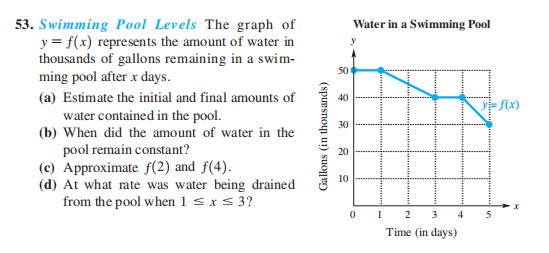### ¿Todavía tienes preguntas de matemáticas?

Pregunte a nuestros tutores expertos
Algebra
Pregunta53. Swimming Pool Levels The graph of Water in a Swimming Pool

$$y = f ( x )$$ represents the amount of water in thousands of gallons remaining in a swim- ming pool after $$x$$ days. (a) Estimate the initial and final amounts of water contained in the pool. (b) When did the amount of water in the pool remain constant? (c) Approximate $$f ( 2 )$$ and $$f ( 4 )$$ . (d) At what rate was water being drained from the pool when $$1 \leq x \leq 3$$ ?

(a) $$50,000$$ gal; $$30,000$$ gal (b) during the first and fourth days (c) $$45,000 ; 40,000$$ (d) $$5000$$ gal per day